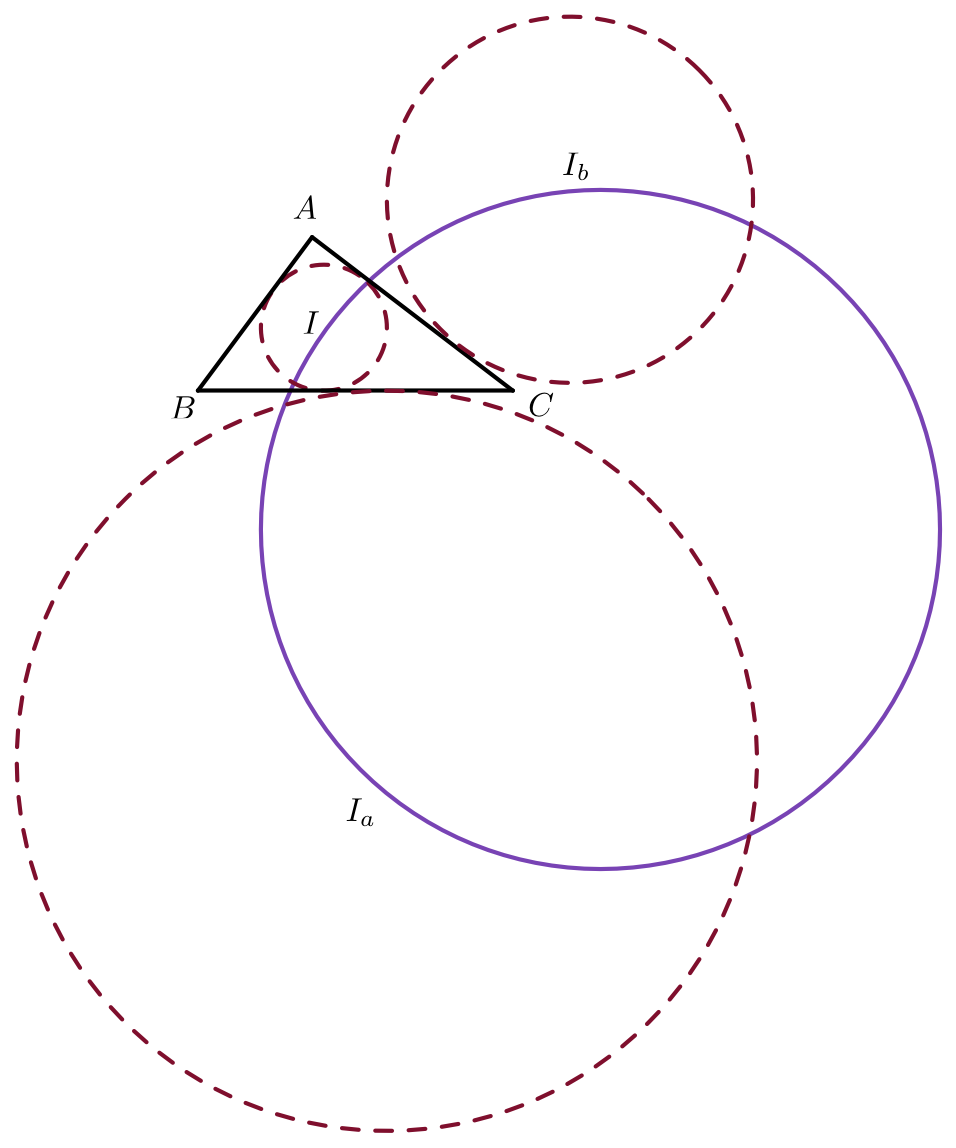# Too Many Circles, Too Distracting

Geometry Level 5Let $I, I_a, I_b$ be the incenter, $A-$ excenter, $B-$ excenter respectively of a triangle $\triangle ABC$ with side lengths $AB= 5, BC= 7, CA= 8.$ Let $R$ be the circumradius of $\triangle II_aI_b.$ Find $\sqrt{3}R.$

×